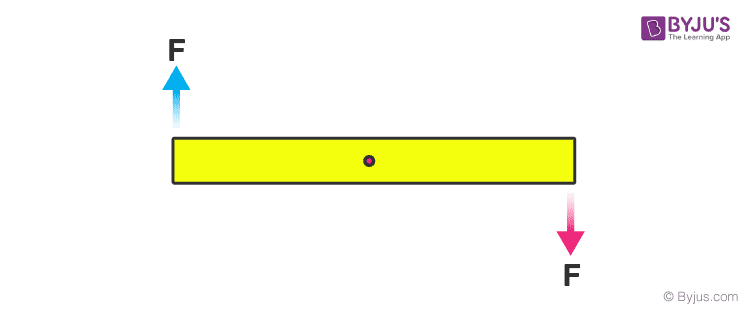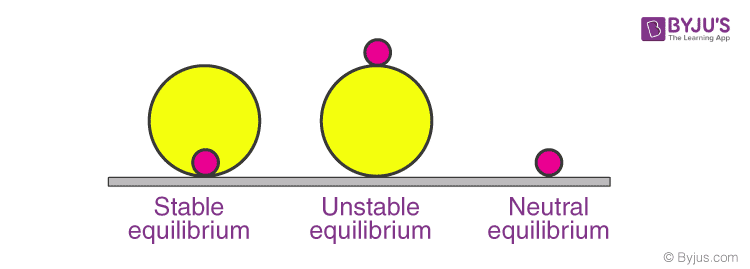# Equilibrium Of Rigid Body

Equilibrium is a state of body where neither the internal energy nor the motion of the body changes with respect to time. Let us try to understand the equilibrium of a rigid body.

If we have to define equilibrium the simplest definition would be it is a point where the net external force, as well as torque acting on the body about COM or any other point, is zero. But to be more specific for a rigid body equilibrium means both rotational and translational equilibrium. For example, consider the following situation:In this case, the body is in translational equilibrium, but the two forces form a couple hence it is not in rotational equilibrium. So suppose for a rod kept on a table minimum number coplanar forces (having a different line of actions) that must act so that body remains in equilibrium? So if we analyse with one force it can’t be either translational or rotational equilibrium, with two force translational is possible but not rotational but with three we can achieve both.

Equilibrium is classified as Dynamic or Static equilibrium. The next question is what static equilibrium is and how it is different from dynamic? So if the body is in equilibrium but continues to move with the uniform velocity it is known as dynamic equilibrium. For example, a ball moving with uniform velocity. On the other hand, if the body is in equilibrium while being at rest it is termed as static equilibrium.

Equilibrium is also classified as stable, unstable and neutral. Let’s see what these mean. A stable equilibrium is one in which is the body is displaced from its equilibrium position then it tends to move towards that equilibrium point. For example, a ball kept at the bottom of a hemisphere. While in case of unstable equilibrium, if it is displaced from that point the body tends to move away from that point. Consider a ball kept at the top of a sphere. If we slide it, the ball tends to roll away from the topmost point.  Similarly, in neutral equilibrium, the body neither moves towards nor away from the equilibrium point. For example, displace a ball kept on a horizontal surface slightly.Stay tuned with BYJU’S for more such interesting articles. Also, register to “BYJU’S – The Learning App” for loads of interactive, engaging Physics-related videos and an unlimited academic assist.